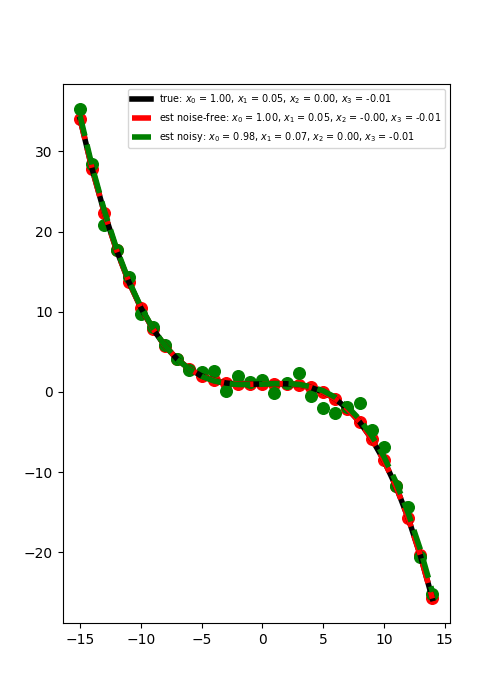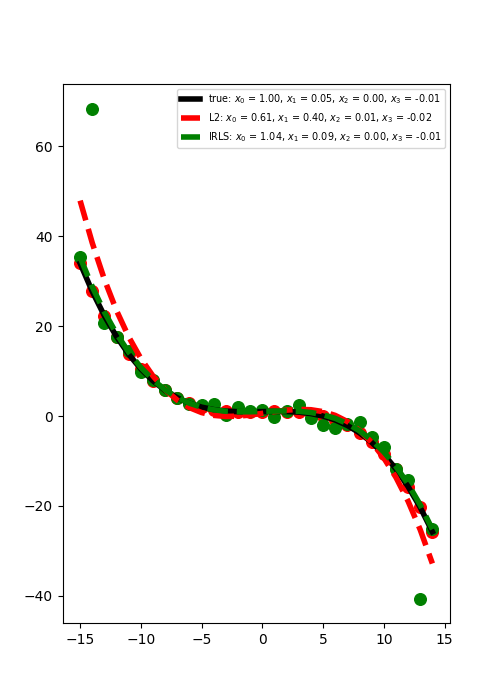# Polynomial Regression¶

This example shows how to use the pylops.Regression operator to perform Polynomial regression analysis.

In short, polynomial regression is the problem of finding the best fitting coefficients for the following equation:

$y_i = \sum_{n=0}^\text{order} x_n t_i^n \qquad \forall i=0,1,\ldots,N-1$

As we can express this problem in a matrix form:

$\mathbf{y}= \mathbf{A} \mathbf{x}$

our solution can be obtained by solving the following optimization problem:

$J= ||\mathbf{y} - \mathbf{A} \mathbf{x}||_2$

See documentation of pylops.Regression for more detailed definition of the forward problem.

import matplotlib.pyplot as plt
import numpy as np

import pylops

plt.close("all")
np.random.seed(10)


Define the input parameters: number of samples along the t-axis (N), order (order), regression coefficients (x), and standard deviation of noise to be added to data (sigma).

N = 30
order = 3
x = np.array([1.0, 0.05, 0.0, -0.01])
sigma = 1


Let’s create the time axis and initialize the pylops.Regression operator

t = np.arange(N, dtype="float64") - N // 2
PRop = pylops.Regression(t, order=order, dtype="float64")


We can then apply the operator in forward mode to compute our data points along the x-axis (y). We will also generate some random gaussian noise and create a noisy version of the data (yn).

y = PRop * x
yn = y + np.random.normal(0, sigma, N)


We are now ready to solve our problem. As we are using an operator from the pylops.LinearOperator family, we can simply use /, which in this case will solve the system by means of an iterative solver (i.e., scipy.sparse.linalg.lsqr).

xest = PRop / y
xnest = PRop / yn


Let’s plot the best fitting curve for the case of noise free and noisy data

plt.figure(figsize=(5, 7))
plt.plot(
t,
PRop * x,
"k",
lw=4,
label=r"true: $x_0$ = %.2f, $x_1$ = %.2f, "
r"$x_2$ = %.2f, $x_3$ = %.2f" % (x, x, x, x),
)
plt.plot(
t,
PRop * xest,
"--r",
lw=4,
label="est noise-free: $x_0$ = %.2f, $x_1$ = %.2f, "
r"$x_2$ = %.2f, $x_3$ = %.2f" % (xest, xest, xest, xest),
)
plt.plot(
t,
PRop * xnest,
"--g",
lw=4,
label="est noisy: $x_0$ = %.2f, $x_1$ = %.2f, "
r"$x_2$ = %.2f, $x_3$ = %.2f" % (xnest, xnest, xnest, xnest),
)
plt.scatter(t, y, c="r", s=70)
plt.scatter(t, yn, c="g", s=70)
plt.legend(fontsize="x-small")Out:

<matplotlib.legend.Legend object at 0x7f4dec2b4550>


We consider now the case where some of the observations have large errors. Such elements are generally referred to as outliers and can affect the quality of the least-squares solution if not treated with care. In this example we will see how using a L1 solver such as pylops.optimization.sparsity.IRLS can drammatically improve the quality of the estimation of intercept and gradient.

# Add outliers
yn += 40
yn[N - 2] -= 20

# IRLS
nouter = 20
epsR = 1e-2
epsI = 0
tolIRLS = 1e-2

xnest = PRop / yn
xirls, nouter, xirls_hist, rw_hist = pylops.optimization.sparsity.IRLS(
PRop,
yn,
nouter,
threshR=False,
epsR=epsR,
epsI=epsI,
tolIRLS=tolIRLS,
returnhistory=True,
)
print("IRLS converged at %d iterations..." % nouter)

plt.figure(figsize=(5, 7))
plt.plot(
t,
PRop * x,
"k",
lw=4,
label=r"true: $x_0$ = %.2f, $x_1$ = %.2f, "
r"$x_2$ = %.2f, $x_3$ = %.2f" % (x, x, x, x),
)
plt.plot(
t,
PRop * xnest,
"--r",
lw=4,
label=r"L2: $x_0$ = %.2f, $x_1$ = %.2f, "
r"$x_2$ = %.2f, $x_3$ = %.2f" % (xnest, xnest, xnest, xnest),
)
plt.plot(
t,
PRop * xirls,
"--g",
lw=4,
label=r"IRLS: $x_0$ = %.2f, $x_1$ = %.2f, "
r"$x_2$ = %.2f, $x_3$ = %.2f" % (xirls, xirls, xirls, xirls),
)
plt.scatter(t, y, c="r", s=70)
plt.scatter(t, yn, c="g", s=70)
plt.legend(fontsize="x-small")Out:

IRLS converged at 4 iterations...

<matplotlib.legend.Legend object at 0x7f4dec7695f8>


Total running time of the script: ( 0 minutes 0.644 seconds)

Gallery generated by Sphinx-Gallery